• Save

Profit Loss and Discount Formula for SSC and Bank Exams

8 months ago 4.8K Views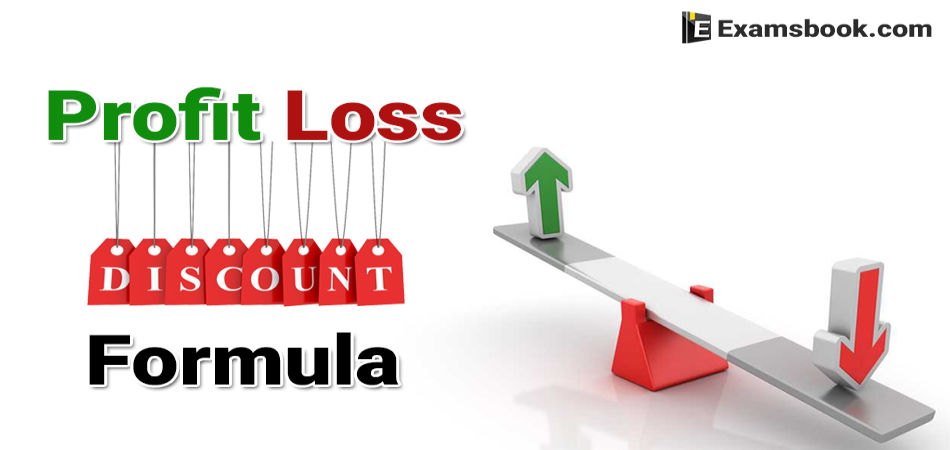Formula is a basic need to solve any question. You will learn about the important formulas associated with profit loss and discount, in this blog. Profit, loss and discount questions are asked every year in bank and SSC exam.

If you have complete knowledge about the formula that how to use formulas, then you can easily solve the questions in the exam. For this purpose, here the questions have been sorted out with formula. So you can remember these formulas and practice the questions.

You can easily solve these type of questions if you know Profit and Loss Trick to solve. Click on Profit-Loss Formulas to know more formulas.

Profit Loss and Discount Formula for Competitive Exams

Cost Price (CP)

The money paid by the shopkeeper to the manufacturer or money paid by the customer to the shopkeeper is called the cost price (CP) of the goods.

Selling Price (SP)

The price at which the shopkeeper sells the goods is called the selling price (SP).

Profit or Gain

Whenever, a person sells an article at price greater than the cost price, he is said to have made a profit or gain.

Profit or Gain = SP – CP [SP>CP]

Loss

If the selling price of an article is less than its cost price, then the shopkeeper suffers a loss.

Loss = CP – SP [CP>SP]

Marked Price (MP)

Basically, to avoid the loss due to bargaining by the customer and get the profit over the cost price trader increase the cost price by a certain value, this increase in value over cost price is known as mark-up and the increased price (i.e., CP +Mark-up) is called the marked price or printed price or list price of the goods.

Marked Price = CP + Mark-up

Marked Price = CP + (Percent mark-up of CP)

Generally, goods are sold at marked price, if there is no further discount, then in this case selling price equals to marked price.

Discount

Discount means concession. It is an offer made by the seller to the buyer for reduction in price to be paid. Basically, it is calculated on the basis of marked price.

Selling price = Marked price (MP) – Discount

Since, marked price = CP+ percent mark-up on CP Remember Mark-up is calculated on the basis of CP, while discount is calculated on the basis of MP.

In general, CP<SP<MP at profit

CP = SP<MP at no profit.

Question based on Mainstream of profit and loss

Q.1. A fruit seller buys orange at the rate of 4 for Rs.10 and an equal number more at 5 for Rs. 10. Sells the whole lot at 9 for Rs. 20. What is his loss or gain percent?

(A) Loss percent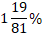(B) Gain percent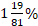(C) No lass or no profit

(D) Loss percent 2%

(a) Let the bought oranges in initial was Rs. x

CP of 1 orange = Rs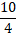CP of x orange =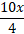According to the case II, CP of 1 orange =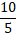CP of x oranges =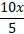CP of 2 x oranges =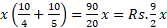SP of 1 orange = Rs. 20/9

SP of 2 oranges = Rs. 40x/9

Loss =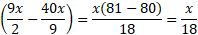Loss Percent =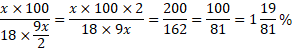Q.2. A dealer offered a machine for sale Rs. 27500, but even if he had charged 10% less, he would have made a profit of 10%. The actual cost of the machine is

(A) Rs. 22000

(B) Rs. 24250

(C) Rs. 22500

(D) Rs. 22275

Let the actual cost of the machine = Rs. x

According to the question,

Question base on MRP and Discount

Q.3. A retailer purchased radio sets at the rate of Rs. 400 each from a wholesaler. He raised the price by 30% and then allowed a discount of 8% on each set. His profit will be

(A) 19%

(B) 78.4%

(C) 22%

(D) 19.6%

Solution (d)

CP of one radio = Rs. 400

According to the question,

Marked Price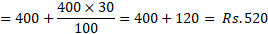Discount =8%

SP of one radio after discount

Per cent profit =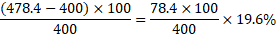Q.4. The cost of manufacture of an article was Rs. 900. The trader wants to gain 25% after giving a discount of 10%. The marked price should be

(A) Rs. 1000

(B) Rs. 1500

(C) Rs. 1250

(D) Rs. 1200

Cost price of the article = Rs. 900

Now, let the market price be Rs. x.

Then, according to the question, 90% of x = 900+25% of 900

⸫  x =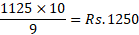Q.5. A grain dealer cheats to the extent of 10% while buying as well as selling by using false weights. His total profit percentage is

(A) 21

(B) 23

(C) 25

(D) 20

Here, x = 10%, y= 10%

⸫ Total profit percentage =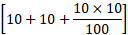=21%

You can ask me in the comment section if you face any problem in these profit loss and discount formulas.